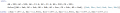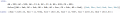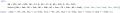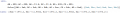# Very basic resistors, but cannot figure them out

#### WBahn

Joined Mar 31, 2012
28,488
Even without doing any derivations, one can tell that the diagonal resistors are low valued and the side resistors are high valued - with approximately two orders of magnitude between the values. Assuming that the side resistors are infinite, then the diagonal resistors are each 11.4 ohms. Assuming that the diagonal resistors are zero, then the side resistors are each 2000 ohms. I think a more accurate guess for the side resistors would be 2000 minus twice the diagonal resistance yielding 1977 ohms.
How are you deciding which resistors are the "diagonal" resistors? Per her diagram, the diagonal resistors would be between A & C and between B & D.

#### WBahn

Joined Mar 31, 2012
28,488
I set up a very simple spreadsheet using the assumption of two low-valued resistors and two high-valued resistors. I did two computations, one for the resistance of the balanced bridge and one for the resistance of the unbalanced bridge.

I then set two control parameters. The first was the value of the low-valued resistor and the other was the ratio of the high-valued resistor to the low-valued resistor.

I then set a goal cell which was the ratio of the unbalanced measurement to the balanced measurement.

I first did a goal seek to find the ratio of the resistors that produced the ratio of the measurements. Then I scaled the low-valued resistor to get the desired measured values.

The whole process took about ten minutes.

As expected, the low-valued resistors need to be just barely larger than the low-valued measurements. The high-valued resistors are right at three times the high-valued measurements.

Joined Nov 5, 2010
215
Isn't the purpose of this to set up six equations with six unknowns? For each node pair you have a resistor, and you make an equation containing it and the variables of the other resistors equaling the measured node resistance. e.g.:

AB = f(Rab, Rbc, Rcd, Rad, Rac, Rbd)

Then you'll be able to solve the six equations and six unknowns algebraically, and then working backwards, plug in the values you start getting into the other equations and eventually produce all the values of the resistors. Then you won't have to depend on any assumptions and you'll be able to show the work. The instructions, after all, were to come up with the equations.

My basic electronic theory is rusty; does anyone have an equation for the network on one of the node pairs?

#### WBahn

Joined Mar 31, 2012
28,488
Isn't the purpose of this to set up six equations with six unknowns? For each node pair you have a resistor, and you make an equation containing it and the variables of the other resistors equaling the measured node resistance. e.g.:

AB = f(Rab, Rbc, Rcd, Rad, Rac, Rbd)

Then you'll be able to solve the six equations and six unknowns algebraically, and then working backwards, plug in the values you start getting into the other equations and eventually produce all the values of the resistors. Then you won't have to depend on any assumptions and you'll be able to show the work. The instructions, after all, were to come up with the equations.

My basic electronic theory is rusty; does anyone have an equation for the network on one of the node pairs?
The problem with setting up the necessary equations is practical. If the bridge is balanced, the equation for the resistance between the two terminals if very straight forward. But if the bridge is unbalanced it becomes quite a mess. There are several ways to go about it. One is to utilize delta-wye transforms. The resulting equation is rather ugly, even with using some intermediate terms (which you wouldn't be able to keep if you then want to invert the relationships and solve for the individual resistances).

I'm guessing here, but I don't think whoever thought up this task actually worked it before assigning it. The case for four resistors is so trivial that I suspect that they thought that adding the other two resistors would be only a modest increase in complexity.

The other possibility is that there are still only four resistors. You can get very close to the measured data (easily within the tolerance of the meter) with just four (even if you constrain them to be two equal pairs of resistors).

#### The Electrician

Joined Oct 9, 2007
2,924
How do the formulas become asymmetrical just because we assume that each of the six resistors is one of two possible values? If they are symmetrical for six different values, how can choosing the same values for some of the resistors break the symmetry that is inherent in the configuration?
Because when they are symmetrical for six different values, there are six degrees of freedom; none of the six resistors is required to be equal to any of the others. The case in this thread where we are assuming there are only two available resistor values is asymmetrical because we have given up some degrees of freedom. It's even more asymmetrical because loss of freedom occurs twice, with different size groups of the constrained resistors; 2 of one value and 4 of the other. One formula gives the value for the two identical resistors and the other formula gives the value for the four identical resistors. The reason there needn't be 4 formulas for the 4 resistors is that we substitute one variable for the 4 that are equal. In other words, we could keep AB, BC, DC and AD as separate and we would have 4 formulas having symmetry of form. If we use AB for BC, DC and AD, we need only one formula to give us the value for the 4 identical resistors. Similarly for the 2 identical resistors.

#### The Electrician

Joined Oct 9, 2007
2,924
As expected, the low-valued resistors need to be just barely larger than the low-valued measurements. The high-valued resistors are right at three times the high-valued measurements.
Three times the high-valued measurements would be 1500 ohms, right? That doesn't work out.

#### RBR1317

Joined Nov 13, 2010
706
Here are the two equations in two unknowns (the two unique values for the side & diagonal resistors) showing the calculation of the equivalent resistance as measured for one high and one low selection. For the resistors forming an unbalanced bridge, a delta-wye transform is performed prior to the series-parallel network simplification. Note that in this equation there is one parallel combination done as the product over sum, and the other parallel combination is done as the reciprocal of the sum of the reciprocals.

#### The Electrician

Joined Oct 9, 2007
2,924
Sometimes one does not realize what should be universally obvious, or not. But given the resistance measurements taken (with a voltmeter), there seemed no other conclusion possible than two resistor values arranged in a square with diagonals.
The problem is equally well satisfied by only four resistors in the "adjacent" positions (no "diagonal" resistors). Their values, starting at the A terminal and proceeding clockwise, are: 988.666 ohms, 11.4657 ohms, 988.666 ohms, 11.4657 ohms.

Sometimes things are not as they seem.

#### RBR1317

Joined Nov 13, 2010
706
Sometimes things are not as they seem.
Seems so simple now! Would that we had started with a circle instead of a tetrahedron.

#### WBahn

Joined Mar 31, 2012
28,488
Three times the high-valued measurements would be 1500 ohms, right? That doesn't work out.
The problem is equally well satisfied by only four resistors in the "adjacent" positions (no "diagonal" resistors). Their values, starting at the A terminal and proceeding clockwise, are: 988.666 ohms, 11.4657 ohms, 988.666 ohms, 11.4657 ohms.
While it's certainly possible that I entered the formulas into my spread sheet incorrectly, I got 1492 Ω for the six resistor case and, using those same formulas but just setting the diagonal resistors to 10e9 Ω back before I made Post #45, I got the same values you did for the four resistor case. I had also sanity checked it by verifying that it produced the correct results for the balanced-bridge case. Unfortunately, I didn't save the spreadsheet and my machine rebooted during the night (I knew it was going to and chose not to save the spreadsheet figuring that I wouldn't need it again).

#### The Electrician

Joined Oct 9, 2007
2,924
I get exactly the same values RBR1317 gets; 9886/5 = 11.4661 ohms for the low valued resistor and 563502/49145 = 1977.2 ohms for the high valued resistor. You are getting 1492 ohms for the high valued resistor. If I substitute that value in the circuit, I get 378.689 ohms for the measurement between adjacent terminals, not the expected 500 ohms. Something went wrong.

#### WBahn

Joined Mar 31, 2012
28,488
I'll reset up the spreadsheet if I get a chance. If I did enter something wrong, I probably won't a second time (or at least not the same thing). The other possibility is that I made a mistake in deriving my equations, so I'll look them over again as well.

Joined Nov 5, 2010
215
I'm a mechanical engineering student, so taking this course which has an electrical component is very hard to understand.

We were given a "black box" with four terminals sticking out of the top, and told to take the voltmeter and do a set of readings for each combination of terminals. 6 combinations total, and they are listed below:
...

Then, the instructions are to find 6 equations that will give you the internal resistors. I have found 4 of those:
...
Please correct me if I'm wrong but I believe the assignment was NOT to come up with the answers, but to write the equations.

#### The Electrician

Joined Oct 9, 2007
2,924
Something that I just noticed is that the 6 resistor solution to this problem is extremely sensitive to the measured values across the adjacent terminals.

I set up a solution to the general problem where all 6 measurements are independent. These images don't show the details of how this was done, but shows a final form where I can easily change measured values and get a numerical solution.

First, here's the solution where the measured values are as the OP gave in post #1, with the 499 values changed to 500:Now restore the two values that were 499 in post #1:The calculated resistor values aren't too much different than when BD and CD are 500.

Now, reset CD to 500 and make BD=500.2, a .04% perturbation:Now the calculated resistor values are wildly different; the diagonal resistors have even become negative! I did a sensitivity analysis and when the values of BD and CD a perturbed together and by the same amount, the sensitivity isn't excessive. But when a single perturbation is applied to AB, AC, BD or CD, the sensitivity is very large. Sensitivity to AD and BC is low.

In the real world, the measurement errors won't be exactly the same for pairs of measurements, so the sensitivity will be high. We won't get the compensation that occurs when the pair AC and AB, or the pair BD and CD, are perturbed together by the same amount.

The OP in this thread saved himself because when he measured AB and AC, he saw that the measurements were both close to 500 ohms (or 499 ohms), and rounded to those exact values so that AB and AC were exactly equal. Had he ended up with, for example, 499.9 ohms for AB and 500.1 ohms for AC, his calculated results would be way off. It makes for a good result if AB and AC are taken to be exactly equal, and similarly for BC and CD; not such a good result if they're slightly different.

Unless the measurements are made with exceptional precision and accuracy, or as the OP did here, the crucial pairs are given exactly equal values, it's unlikely that the calculated results will be very close to the actual resistors in the box.

Just for a final exhibit, look what we get if both crucial pairs are 499 ohms:#### The Electrician

Joined Oct 9, 2007
2,924
Please correct me if I'm wrong but I believe the assignment was NOT to come up with the answers, but to write the equations.
You're quite right. I've been waiting for the OP to respond to my demonstration in post #32 that the equations he had developed to that point were giving wrong results. When he didn't respond, the rest of us just got carried away. I wonder if he has gone away, as often happens.

#### WBahn

Joined Mar 31, 2012
28,488
You're quite right. I've been waiting for the OP to respond to my demonstration in post #32 that the equations he had developed to that point were giving wrong results. When he didn't respond, the rest of us just got carried away. I wonder if he has gone away, as often happens.
I'm guessing that it's a "she" (based on the name Ilana, but that is just a gut impression).

•dapEK

#### dapEK

Joined Oct 7, 2015
15
I somehow didn't receive any email notifications so assumed this thread went dead. I also ran the numbers using my formulas, and came up with the same 255 and 11.4 variations mentioned above. I've since corrected my formulas for the diagonal to read:

But, I received a sample from a classmate of what he thinks these equations should be, and I am absolutely perplexed as to his theory of them. I asked him why he did such things, and he doesn't know. He got them from a friend who I presume got them from a friend. See attached below:

http://imgur.com/ZLZg0UF

#### dapEK

Joined Oct 7, 2015
15
But, I've been reading through all of your posts and it seems like this assignment isn't as well thought out as they had planned, there seems to be a lot of underlying theory that the professor didn't think about. What you're talking about is LEVELS over what we're doing. To give you an idea, other experiments in this set of experiments included:
1.finding the value of resistors and measuring them to compare,
2. using a voltage divider and seeing if Ohm's law held, and
3. playing with an oscilloscope, for no real reason

I seriously doubt it was supposed to be this complicated, but I didn't understand the theory and still don't, and that's vexing me.

However, I strongly believe that there are only TWO unique resistors inside this box, and there's a good chance there are no diagonals. I agree with the previous poster who said that four resistors would satisfy the equations, not six.

#### JoeJester

Joined Apr 26, 2005
4,390
The schematic in post number 33 will work and give the appropriate results using E192 resistors.